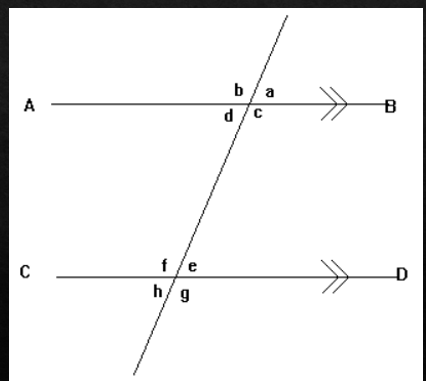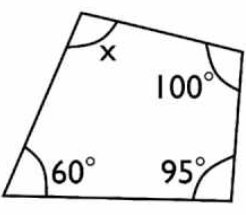Angle Relationships
Find the Missing Angle
Triangle Sum Theorem
Vocabulary
100

Two angles are corresponding. The measure of the first angles is 42 degrees, what is the measure of the second angle?

42 degrees

100

What is the complement of an angle that measures 12 degrees?

78 degrees

100

Is it possible to have two right angles in a triangle? Why or why not?

No, because two right angles add up to 180 degrees.

100

A rectangle has 4 angles. What is the total sum of these angles?

360 degrees

100

What type of triangle has two congruent sides?

Isosceles Triangle

200

If angle 1 and 2 are supplementary and the measure of angle 1 is 112, what is the measure of angle 2?

68 degrees

200

What is the supplement of an angle that measures 99 degrees?

81 degrees

200

In Triangle DEF, the measure of angle E is 30, angle F is a right angle, what is the measure of angle D?

60 degrees

200

A trapezoid has an angle of 129°, another angle of 51°, and another angle of 129°. What is the angle of the 4th angle?

51 degrees

200

What is the name of a triangle with three equal angles?

Equiangular Triangle

300

If angle 1 and 2 are both complementary, what is the measure of angle 1 if angle 2 measures 32 degrees?

58 degrees

300

An isosceles triangle has an angle of 90°. What are the angles of the other 2 angles?

45 and 45 degrees

300Find angle x and angle y.

angle x = 113 and angle y = 67

300

A four-sided polygon has an angle of 40°, 80°, and 90°. What is the angle of the 4th angle?

150 degrees

300

What is the name of an angle that is 180 degrees.

Straight Angle

400

Angle A = 94°. Angle B is adjacent to angle A. What is the measure of angle B?

86 degrees

400Angle d is 60°, what is the angle measure of angles e and f?

angle e = 60° and angle f = 120°

400

In Triangle MNO, if angle M is 3x, angle N is 2x+40, and angle I is x-20, what is the sum of the three angles?

180 degrees

400

Can a quadrilateral have 2 obtuse angles?

yes

400

What is the relationship of two angles that add up to 180?

Supplementary Angles

500

Name the four angle relationships that tell us the angles are congruent.

vertically opposite, corresponding angles, alternate interior angles, alternate exterior angles

500

Angle A and B are complementary. Angle A and Angle B are equal. What are the angle measures of both angles?

Angle A is 45 degrees. Angle B is 45 degrees.

500

X=5. In Triangle MNO, if angle M is (40 + X), angle N is (75 - X), what is the angle of angle O?

65 degrees

500Find x

x = 105

500

What is a reflex angle?

Angle between 180° and 360°

Click to zoom